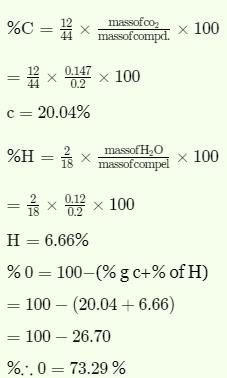Courses

# Test: Chemistry Mock- 7

## 45 Questions MCQ Test NEET Mock Test Series | Test: Chemistry Mock- 7

Description
This mock test of Test: Chemistry Mock- 7 for NEET helps you for every NEET entrance exam. This contains 45 Multiple Choice Questions for NEET Test: Chemistry Mock- 7 (mcq) to study with solutions a complete question bank. The solved questions answers in this Test: Chemistry Mock- 7 quiz give you a good mix of easy questions and tough questions. NEET students definitely take this Test: Chemistry Mock- 7 exercise for a better result in the exam. You can find other Test: Chemistry Mock- 7 extra questions, long questions & short questions for NEET on EduRev as well by searching above.
QUESTION: 1

Solution:
QUESTION: 2

Solution:
QUESTION: 3

### Which one of the following compounds is prepared in the laboratory from benzene by substitution reaction?

Solution:
QUESTION: 4
An electron is moving in Bohr's fourth orbit. Its de Broglie wavelength is λ. What is the circumference of the fourth orbit?
Solution:
QUESTION: 5
The units nanometer, fermi, angstrom and attometre arranged in decreasing order
Solution:
QUESTION: 6
When glucose reacts with bromine water, the main product is
Solution:
QUESTION: 7
Which of the following arrangement of molecules is correct on the basis of their dipole moments?
Solution:
QUESTION: 8

For an ideal gas the relationship between internal energy change .(∆E) and enthalpy change(∆H)is

Solution: Its Delta H=Delta E+ Delta(P×V) so option A  is correct.
QUESTION: 9
when a catalyst is added to a reversible reaction in equilibrium state, the value of equilibrium constant
Solution:
QUESTION: 10

The rate of chemical reaction is doubled for every 10oC rise in temperature because of

Solution:
QUESTION: 11
Internal energy of a substance/system is a
Solution:
QUESTION: 12
Amoxillin is semi-synthetic modification of
Solution:
QUESTION: 13
How many isomers (including stereisomers )could be obtained by replacing one hydrogen of propene with chlorine ?
Solution:
QUESTION: 14

The atomic radius of elements of which of these would be nearly same

Solution:

The atomic radii of the d- block elements of a given series decrease with increase in atomic number but this decrease becomes small after midway. The decrease in atomic radii in the beginning, is due to an increase in nuclear charge from member to member which tends to reduce the size. At the same time, the addition of extra electrons to (n-1)d orbitals also provides the screening effect. As the number of d-electrons increases, the screening effect increases. Thus, there are operating two effects namely screening effect and nuclear charge effect which oppose each other. In the midway onwards of the series both these effects become nearly equal and thus, there is no change in atomic radii inspite of the fact that atomic number increases gradually.

QUESTION: 15
Ce-58 is a member of
Solution:
QUESTION: 16

Which one of the following cyano complexes would exhibit the lowest value of paramagnetic behaviour?
(At. No. : Cr = 24, Mn = 25, Fe = 26, Co = 27)

Solution:
QUESTION: 17
In alkaline medium ClO₂ oxidises H₂O₂ to O₂ and itself gets reduced to Cl⁻.How many moles of H₂O₂ are oxidised by 1 mole of ClO₂ ?
Solution:
QUESTION: 18
Which among the following metals belong to the same group of the periodic table?
Solution:
QUESTION: 19
Pressure cooker reduces cooking time because
Solution:
QUESTION: 20
The metal extracted by electrolysis of its fused salt is
Solution:
QUESTION: 21
The chief ore of a aluminium is
Solution:
QUESTION: 22
The components present in producer gas are
Solution:
QUESTION: 23
When excess of water is added to BiCl₃ solution
Solution:
QUESTION: 24
The chemical reaction between Na₂S₂O₃ and I₂ in aq. medium produces
Solution:
QUESTION: 25
Bleaching powder is obtained by the action of chlorine gas and
Solution:
QUESTION: 26
Blanc fixe used in paints is
Solution:
QUESTION: 27
In Wurtz reaction the reagent used is
Solution:
QUESTION: 28

Hardness of water is due to which pair of ions ?

Solution:
QUESTION: 29
A white salt is readily soluble in water and gives a colourless solutions with a pH of about 9.The salt would be
Solution:
QUESTION: 30

An alkane forms isomers if minimum number of C-atom is :

Solution:

For an alkane having isomers, the minimum no of carbon atoms is 4. This will give two isomers as n-butane and iso-butane.

QUESTION: 31
Grignard reagent is not prepared in aqueous medium but prepared in ether medium, because
Solution:
QUESTION: 32
What is formed,when (CH₃)₄N.OH is heated ?
Solution:
QUESTION: 33
Which of the following is the strongest acid?
Solution:
QUESTION: 34

Which of the following organometallic compound is σ and π bonds?

Solution:
QUESTION: 35

Which of the following compounds can react with the Lewis base Cl in a Lewis acid-base reaction?

Solution:
QUESTION: 36
The highest electronegative element is
Solution: The electronegativity values for the elements decrease down a group. This is because as we move down the group atomic radius as well as screening effect increases. Thus, the force of attraction with which the nucleus pulls the valence electrons towards itself decreases and consequently the electronegativity values also decrease. The electronegativity values for alkali metals follows the order:
Li > Na > K > Rb.
QUESTION: 37
Caprolactam polymerises to give
Solution:
QUESTION: 38

On combustion, 0.2g of an organic compound containing carbon, hydrogen and oxygen yields 0.147 g carbon dioxide and 0.12 g water. The percentage of carbon, hydrogen and oxygen respectively in the compound are

Solution:QUESTION: 39

Oxidation number of S in S₂O₃2⁻ is

Solution:
QUESTION: 40
Potassium crystallizes with a
Solution:
QUESTION: 41
Which of the following is a colligative property ?
Solution:
QUESTION: 42
The optical isomerism is shown by :
Solution:
QUESTION: 43
A balanced chemical reaction is in accordance with
Solution:
QUESTION: 44
Gold number gives
Solution:
QUESTION: 45

Which of the following is paramagnetic ?

Solution:

Cl- is a weak field ligand and it does not cause the pairing of unpaired 3d electrons. Hence, [NiCl4]2- is paramagnetic.